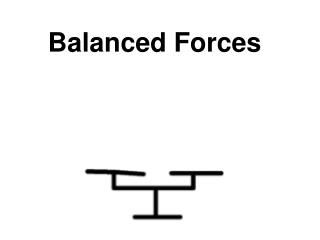Download PresentationBalanced ForcesBalanced Forces - PowerPoint PPT Presentation

Download PresentationBalanced Forces
An Image/Link below is provided (as is) to download presentation

Download Policy: Content on the Website is provided to you AS IS for your information and personal use and may not be sold / licensed / shared on other websites without getting consent from its author. While downloading, if for some reason you are not able to download a presentation, the publisher may have deleted the file from their server.

- - - - - - - - - - - - - - - - - - - - - - - - - - - E N D - - - - - - - - - - - - - - - - - - - - - - - - - - -
Presentation Transcript

1. Balanced Forces

2. Levers

3. Write out the statements that are true. • a The longer the lever, the bigger the force that is needed to move an object. • b It is easier to close a door if you push the door close to the hinge • c The shorter the lever, the bigger the force that is needed to move an object • d Joints are examples of pivots. • e Bones are examples of levers.

4. C, D and E

5. Learning Objective To investigate, through practical experimentation, the principle of moments.

6. Recording your results • What do we need to record? • How many columns will we need in our table?

7. Recording your results

8. Weight and Mass • YouTube - Eureka! Episode 7 - Weight vs. Mass YouTube - Eureka! Episode 6 - Gravity Racing Balls

9. Write out each term along with its correct description Descriptions • anticlockwise moments = clockwise moments • two boys of different weights sit opposite each other on a see saw, both the same distance from the pivot • the turning effect of a force unbalanced system moment balanced system Lever Principle GCSE PHYSICS: Moments

10. Moment calculation 0.5m 500N pivot Gina weighs 500N and stands on one end of a seesaw. She is 0.5m from the pivot. What moment does she exert? moment = 500 x 0.5 = 250 Nm

11. Moment equation moment = force (N) x distance from pivot (cm or m) moment f x d The moment of a force is given by the equation: Moments are measured in Newton centimetres (Ncm) or Newton metres (Nm).

12. Principle of moments The girl on the left exerts an anti-clockwise moment, which equals... The girl on the right exerts a clockwise moment, which equals... her weight x her distance from pivot her weight x her distance from pivot

13. Principle of moments If the anticlockwise moment and clockwise moment are equal then the see-saw is balanced. This is known as the principle of moments. When something is balanced about a pivot: total clockwise moment = total anticlockwise moment

14. Principle of moments – calculation Two girls are sitting on opposite sides of on a see-saw. One girl weighs 200N and is 1.5m from the pivot. Where must her 150N friend sit if the seesaw is to balance? When the see-saw is balanced: total clockwise moment = total anticlockwise moment 200N x 1.5m = 150N x distance 200 x 1.5 = distance 150 distance of second girl = 2m

15. Anagrams

16. trolley load arm counterweight loading platform tower Why don’t cranes fall over? Tower cranes are essential at any major construction site. Concrete counterweights are fitted to the crane’s short arm. Why are these needed for lifting heavy loads?

17. 3m 6m 10,000N Why don’t cranes fall over? Using the principle of moments, when is the crane balanced? ? moment of = moment of load counterweight If a 10,000N counterweight is three metres from the tower, what weight can be lifted when the loading platform is six metres from the tower?

18. counterweight x moment of counterweight distance of counterweight from tower = = 10,000 x 3 = 30,000 Nm moment of load load x distance of load from tower = = ? x 6 moment of load = moment of counterweight ? x 6 = 30,000 ? = 3,000 6 ?= 5,000 N Why don’t cranes fall over?

19. Crane operator activity Where should the loading platform be on the loading arm to carry each load safely?